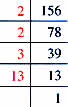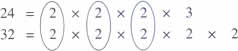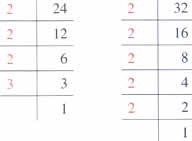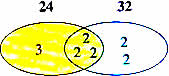Friday , August 19 2022# NCERT 5th Class (CBSE) Mathematics: Factors And Multiples

## PRIME FACTORIZATION

All composite numbers can be broken up into their factors.

When the factors of a number are all prime numbers, it is called the prime factorisation of the number.

There are two ways of finding the prime factors of a number.

### (a) Prime Factorization Method

56 = 2×28
= 2×2×14
= 2×2×2×7

The prime factors of 56 = 2×2×2×7.

### (b) Division MethodThe prime factors of 156 = 2×2×3×13

## EVEN AND ODD NUMBERS

A numbers that is a multiple of 2 is called an even number. All even number and with the digits 0, 2, 4, 6, or 8.

A number that is not a multiple of 2 is called and odd number. All odd numbers end with the digits 1, 3, 5, 7, or 9.

The product of an even number and any other number is always even. The product of two odd numbers is always odd.

## MORE DIVISIBILITY RULES

4 If the number formed by its last digits is divisible by 4 or are Zeroes.
For example, 2832, 916, 83001

6 If the number is divisible by both 2 and 3.
For example, 384, 1602, 2982

8 If the number formed by its last three digits is divisible by 8.
For example, 7112, 13760, 26584

11 If the difference between the sum of digits in odd places and sum of digits in the even places is 0 or divisible by 11.

For example, 9845

9                       8                    4                        5
↓                       ↓                     ↓                         ↓
Odd place      Event place      Odd place      Event place

9+4 = 13
8+5 = 13
Difference = 0

## HIGHEST COMMON FACTOR

Let us look at the common factors of 12 and 18.

Factors of 12: 1, 2, 3, 4, 6, 12

Factors of 18: 1, 2, 3, 6, 9, 18

The common factors of 12 and 18 are 1, 2, 3, and 6

The Highest Common Factor (HCF) of 12 and 18 is 6.

The HCF of two numbers is the greatest number that can divide both numbers without leaving any remainder.

So, in the above example, 6 is the greatest number that can divide both 12 and 18 without leaving a reminder.

### CHECKPOINT

The HCF of two or more numbers is always smaller than or equal to the numbers.

There are three methods used to find the HCF of numbers.

### 1. Prime Factorization Method

Find the HCF of 24 and 32 using the prime factorization method.2×2×2 = 8HCH of 24 and 32 is 8.

Prime Factors of 24

2      2

2      2

Prime Factors of 32

2                  2
2         2       2

Common prime Factors of 24 and 32HCF of 24 and 32 = 2×2×2 = 8

## चुनौती हिमालय की 5th NCERT CBSE Hindi Rimjhim Ch 18

चुनौती हिमालय की 5th Class NCERT CBSE Hindi Book Rimjhim Chapter 18 प्रश्न: लद्दाख जम्मू-कश्मीर राज्य में …